# RD Sharma Class 10 Ex 15.2 Solutions Chapter 15 Areas Related To Circles

In this chapter, we provide RD Sharma Class 10 Ex 15.2 Solutions Chapter 15 Areas Related To Circles for English medium students, Which will very helpful for every student in their exams. Students can download the latest RD Sharma Class 10 Ex 15.2 Solutions Chapter 15 Areas Related To Circles pdf, Now you will get step by step solution to each question.

# Chapter 15: Areas Related To Circles Exercise – 15.2

### Question: 1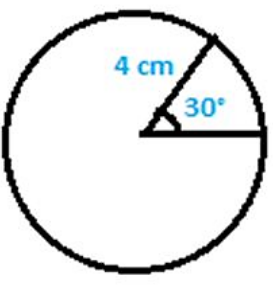Find in terms of π, the length of the arc that subtends an angle of 30 degrees, at the center of O of the circle with a radius of 4 cm.

### Solution:

Given Data:

Angle subtended at the centre ‘O’ = 30°

Formula to be used: Length of arc = θ/360 × 2πr cm

Length of arc = 30/360 × 2π∗4 cm = 2π/3 cm

Therefore, the Length of arc the length of the arc that subtends an angle of 60 degrees is 2π/3 cm

### Question: 2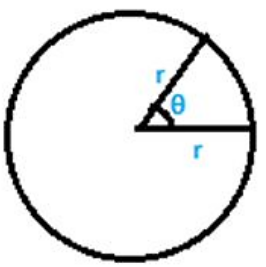Find the angle subtended at the centre of circle of radius 5 cm by an arc of length 5π/3 cm.

### Solution:

Given data: Radius = 5 cm

Length of arc = 5π/3 cm

Formula to be used: Length of arc = θ/360 ∗ 2πr cm

5π/3 cm = θ/360 ∗ 2πr cm

Solving the above equation, we have:

θ = 60°

Therefore, angle subtended at the centre of circle is 60°

### Question: 3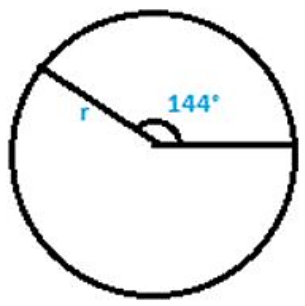An arc of length cm subtends an angle of 144° at the center of the circle.

### Solution:

Given Data:

Length of arc = 25 cm

θ = Angle subtended at the centre of circle = 144°

Formula to be used:

Length of arc = θ/360 ∗ 2πr cm

θ/360 ∗ 2πr cm = 144/360 east 2πr cm = 4π/5 ∗ r cm

As given in the question, length of arc = cm,

Therefore, cm = 4π/5 ∗ r cm

Solving the above equation, we have r = 25 cm.

Therefore the radius of the circle is found to be 25 cm.

### Question: 4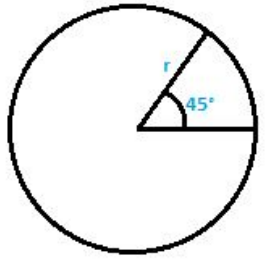An arc of length 25 cm subtends an angle of 55° at the center of a circle. Find in terms of radius of the circle.

### Solution:

Given Data:

Length of arc = 25 cm

θ = Angle subtended at the centre of circle = 55°

Formula to be used:

Length of arc = θ/360 ∗ 2πr cm

= 55/36 ∗ 2πr cm

As given in the question length of arc = 25 cm, hence,

25 cm = 55/360 ∗ 2π ∗ r cm

25 = 11πr/36

Therefore, the radius of the circle is 900/11π

### Question: 5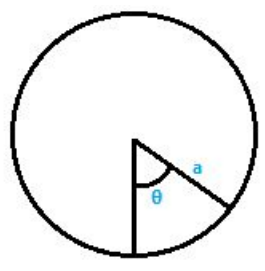Find the angle subtended at the center of the circle of radius ‘a’ cm by an arc of π/4 length cm.

### Solution:

Given data:

Length of arc = aπ/4 cm

θ = angle subtended at the centre of circle

Formula to be used:

Length of arc = θ/360 ∗ 2πr cm

Length of arc = θ/360 ∗ 2πa cm

θ/360 ∗ 2πa cm = aπ/4 cm

Solving the above equation, we have

θ = 45°

Therefore, the angle subtended at the centre of circle is 45°

### Question: 6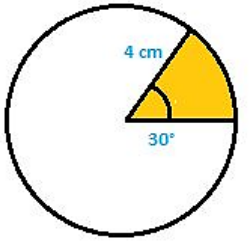A sector of the circle of radius 4 cm subtends an angle of 30°. Find the area of the sector.

### Solution:

Given Data: Radius = 4 cm

Angle subtended at the centre ‘O’ = 30°

Formula to be used:

Area of the sector = θ/360 ∗ πr2

Area of the sector = 30/360 ∗ π42

Solving the above equation, we have: Area of the sector = 4.9 cm

Therefore, Area of the sector is found to be 4.9 cm2

### Question: 7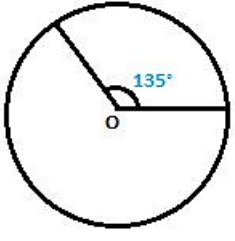A sector of a circle of radius 8 cm subtends an angle of 135. Find the area of sector.

### Solution:

Given Data: Radius = 8 cm

Angle subtended at the centre ‘O’ = 135°

Formula to be used:

Area of the sector = θ/360 ∗ πr2

Area of the sector = 135/360 ∗ π82

= 528/7 cm2
Therefore, Area of the sector calculated is 528/7 cm2

### Question: 8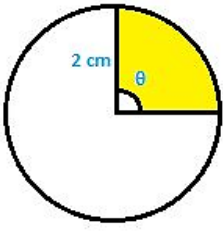The area of sector of circle of radius 2 cm is cm2. Find the angle subtended by the sector.

### Solution:

Given Data: Radius = 2 cm

Angle subtended at the centre ‘O’

Area of sector of circle = cm2

Formula to be used:

Area of the sector = θ/360 ∗πr2

Area of the sector = θ/360 ∗ π32

= πθ/90

As given in the question area of sector of circle = cm2

cm= π θ/90

Solving the above equation, we have

θ = 90°

Therefore, the angle subtended at the centre of circle is 90°

### Question: 9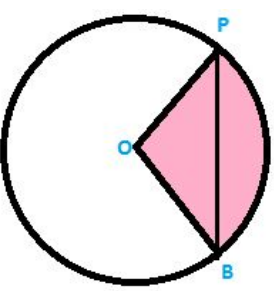PQ is a chord of circle with centre ‘O’ and radius 4 cm. PQ is of the length 4 cm. Find the area of sector of the circle formed by chord PQ.

### Solution:

Given Data:

PQ is chord of length 4 cm. Also, PO = QO = 4 cm OPQ is an equilateral triangle.

Angle POQ = 60°

Area of sector (formed by the chord (Shaded region)) = (Area of sector)

Formula to be used:

Area of the sector = θ/360 ∗ πr2

Area of the sector = 60/360 ∗ π42 = 32π/3

Therefore, Area of the sector is 32π/3 cm2

### Question: 10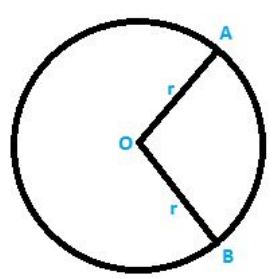In a circle of radius 35 cm, an arc subtends an angle of 72° at the centre. Find the length of arc and area of sector.

### Solution:

Given Data: Radius = 35 cm

Angle subtended at the centre ‘O’ = 72°

Area of sector of circle =?

Formula to be used: Length of arc = θ/360 ∗ 2πr cm

Length of arc = 108/360 ∗ 2πx = 42 cm

Solving the above equation we have,

Length of arc = 44 cm

We know that,

Area of the sector = θ/360 ∗ πr2

Area of the sector = 72/360 ∗ π352

Solving the above equation, we have,

Area of the sector = (35 × 22) cm2

Therefore, Area of the sector is 770 cm2

### Question: 11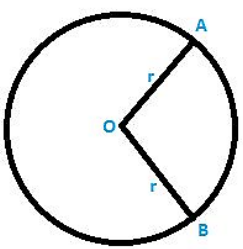The perimeter of a sector of a circle of radius 5.7 m is 27.2 m. find the area of the sector.

### Solution:

Given Data:

Radius = 5.7 cm = OA = OB [from the figure shown above]

Perimeter = 27.2 m

Let the angle subtended at the centre be θ

Perimeter = θ/360 ∗ 2πr cm + OA + OB

= θ/360 ∗ 2πx 5.7 cm + 5.7 + 5.7

Solving the above equation we have,

θ = 158.8°

We know that, Area of the sector = θ/360 ∗ πr2

Area of the sector = 158.8/360 ∗ π 5. 72

Solving the above equation we have,

Area of the sector = 45.048 cm2

Therefore, Area of the sector is 45.048 cm2

### Question: 12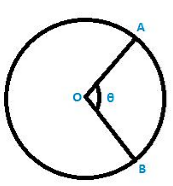The perimeter of a certain sector of a circle of radius is 5.6 m and 27.2 m. find the area of a sector.

### Solution:

Given data:

Radius of the circle = 5.6 m = OA = OB

(AB arc length) + OA + OB = 27.2

Let the angle subtended at the centre be θ

We know that,

Length of arc = θ/360 ∗ 2πr cm

θ/360 ∗ 2πr cm + OA + OB = 27.2 m

θ/360 ∗ 2πr cm + 5.6 + 5.6 = 27.2 m

Solving the above equation, we have,

θ = 163.64°

We know that, Area of the sector = θ/360 ∗ πr2

Area of the sector = 163.64/360 ∗ π 5.62

On solving the above equation, we have,

Area of the sector = 44.8 cm2

Therefore,

Area of the sector is 44.8 cm2

### Question: 13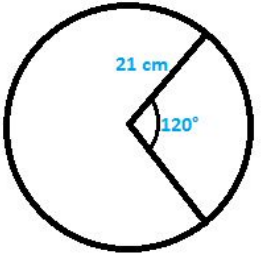A sector was cut from a circle of radius 21 cm. The angle of sector is 120°. Find the length of its arc and its area.

### Solution:

Given data: Radius of circle (r) = 21 cm

θ = angle subtended at the centre of circle = 120°

Formula to be used:

Length of arc = θ/360 ∗ 2πr cm

Length of arc = 120/360 ∗ 2πx 21cm

On solving the above equation, we get, Length of arc = 44 cm

We know that, Area of the sector = θ/360 ∗ πr2

Area of the sector = 120/360 ∗ π212

Area of the sector = (22 × 21) cm2

Therefore, Area of the sector is 462 cm2

### Question: 14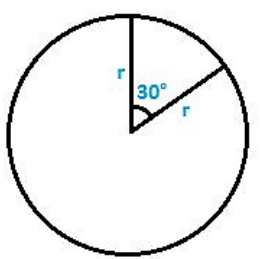The minute hand of a circle is √21 cm long. Find the area described by the minute hand on the face of clock between 7:00 a.m to 7:05 a.m.

### Solution:

Given data: Radius of the minute hand (r) = √21cm

Time between 7: 00 a. m to 7: 05 a. m = 5 min

We know that, 1 hr = 60 min, minute hand completes

One revolution = 360° 60 min = 360°

θ = Angle subtended at the centre of circle = 5 × 6° = 30°

Area of the sector = θ/360 ∗ πr2

Area of the sector = 30/360 ∗ pi352

Area of the sector = 5.5 cm2

Therefore, Area of the sector is 5.5 cm2

### Question: 15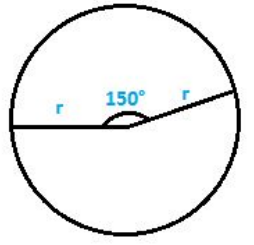The minute hand of clock is 10 cm long. Find the area of the face of the clock described by the minute hand between 8 a. m to 8:25 a.m.

### Solution:

Given data: Radius of the circle = radius of the clock = length of the minute hand = 10 cm

We know that, 1 hr = 60 min 60 min = 360° 1 min = 6°

Time between 8:00 a. m to 8:25 a. m = 25 min

Therefore, the subtended = 6° × 25 = 150°

Formula to be used:

Area of the sector = θ/360 ∗ πr2

Area of the sector = 150/360 ∗ π102

Area of the sector = 916.6 cm= 917 cm2

Therefore, Area of the sector is 917 cm2

### Question: 16

A sector of 56° cut out from a circle subtends area of 4.4 cm2. Find the radius of the circle.

### Solution:

Given data: Angle subtended by the sector at the centre of the circle,

θ = 56°

Let the radius of the circle be = r cm

Formula to be used:

Area of the sector = 56/360 ∗ πr2

On solving the above equation, we get, r= √(9/1) cm

r = 3 cm

Therefore, radius of the circle is r = 3 cm

### Question: 17

In circle of radius 6 cm. Chord of length 10 cm makes an angle of 110° at the centre of circle. Find:

(i) Circumference of the circle

(ii) Area of the circle

(iii) Length of arc

(iv) The area of sector

### Solution:

Given data: Radius of the circle = 6 cm

Chord of length = 10 cm

Angle subtended by chord with the centre of the circle = 110°

Formulae to be used: Circumference of a circle = 2

Area of a Circle = Area of the sector = θ/360 ∗ πr2

Length of arc = 90/360 ∗ 2πx 28cm

Circumference of a circle = 2

= 2 × 3.14 × 8 = 37.7 cm

Area of a Circle = 3.14 × 6 × 6 = 113.14 cm2

Area of the sector = θ/360 ∗ πr/2

Area of the sector = 110/360 ∗ π62

On solving the above equation we get,

Area of the sector = 33.1 cm2

Length of arc = θ/360 ∗ 2πr cm [latex]

Length of arc = 110/360 2π6cm

On solving the above equation we get, Length of arc = 22.34 cm.

Therefore, Circumference = 37.7 cm

Area of a Circle = 113.14 cm2

Area of the sector = 33.1 cm2

### Question: 18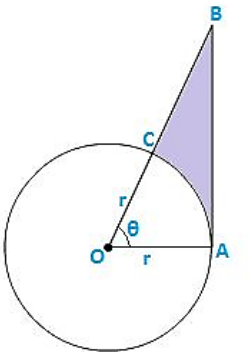The given figure shows a sector of a circle with centre ‘O’ subtending an angle θ°. Prove that:

1. Perimeter of shaded region is

2. Area of the shaded region is

### Solution:

Given Data: Angle subtended at the centre of the circle = θ°

Angle OAB = 90° [at point of contact, tangent is perpendicular to radius]

OAB is a right angle triangle

cos θ = adjside/hypotenuse = r/OB = OB = r sec θ

sec θ = opposite/adjside = AB/r = AB = r tan θ

Perimeter of the shaded region = AB + BC + CA (arc)

=  r tan? + (OB – OC) + θ/360 ∗ 2πr cm

= r(tan θ + sec θ + π θ/180 − 1)

Area of the shaded region = (Area of triangle AOB) – (Area of sector)

(1/2 ∗ OA ∗ AB) − θ/360 ∗ πr2

On solving the above equation we get, r2/2[tan θ − πθ/180]

### Question: 20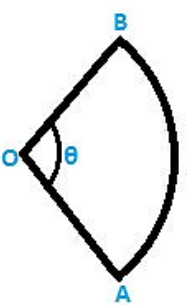The diagram shows a sector of circle of radius ‘r’ cm subtends an angle θ. The area of sector is A cm2 and perimeter of sector is 50 cm. Prove that θ = 360/π (25/r – 1) and A = 25 r  r2

### Solution:

Given Data: Radius of circle = ‘r’ cm

Angle subtended at centre of the circle = θ

Perimeter = OA + OB + (AB arc)

As given in the question, perimeter = 50

Area of the sector = θ/360 ∗ πr2

On solving the above equation, we have A = 25r – r2

Hence, proved.

All Chapter RD Sharma Solutions For Class10 Maths

I think you got complete solutions for this chapter. If You have any queries regarding this chapter, please comment on the below section our subject teacher will answer you. We tried our best to give complete solutions so you got good